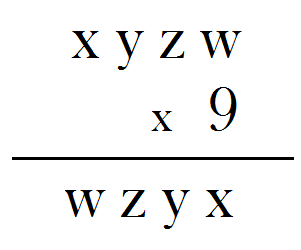# Going Reverse!Mohan was scribbling random four-digit numbers and multiplying them by 9. One particular four-digit number caught his attention. When he multiplied this number by 9, the result was again a four-digit number; curiously, the resulting number was reverse of the original number.

Let me elaborate on this so that you don't get me wrong. Suppose the original number was xyzw (x, y, z, w are not necessarily distinct), then upon multiplying xyzw by 9, the resulting number is wzyx. I can't give a true example because that will be giving away the answer; but, suppose the original number was 1234, then 1234 x 9 should give 4321. Of course, 1234 x 9 = 11106; now, 11106 is neither 4321 nor is it a four-digit number. So, 1234 is not the number Mohan hit upon.

Can you find the four-digit number which when multiplied by 9 yields a four-digit number as the result and which is the reverse of the original number?

Some useful links for

• Union Public Service Commission - www.upsc.gov.in
• IIT-Kharagpur - www.iitkgp.ac.in
• Indian Statistical Institute - www.isical.ac.in
• Indian Institute of Technology Madras - www.iitm.ac.in
• Indian Institute of Management, Ahmedabad - www.iimahd.ernet.in
• Indian Institute of Mass Commission - www.iimc.nic.in
• IIT Bombay - www.iitb.ac.in
• Indian School of Mines, Dhanbad - www.ismdhanbad.ac.in
• Birla Institute of Technology, Ranchi - www.bitmesra.ac.in
• Central Institute of Fisheries Nautical and Engineering Training - www.cifnet.nic.in
• Indian Institute of Information Technology, Allahabad (Deemed University) - www.iiita.ac.in
• Central Marine Fisheries Research Institute, Kochi - www.cmfri.com
• Tata Institute of Social Sciences, Mumbai - www.tiss.edu